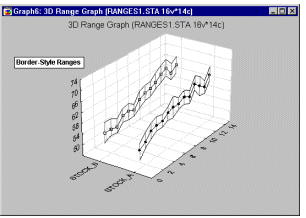## How to plot 3D box plot using SAS?

Hello everybody,

I want to plot a 3D box blot which illustrates means with standard error bars from calculated data as I show bellow:3D Box Plot

All of data, which I have, are numeric type.

How can I do that?

1 ACCEPTED SOLUTION

Accepted Solutions

## Re: How to plot 3D box plot using SAS?

I have a suggestion, However it is not the best one and needs to be corrected.

``````/* Set the graphics environment */
goptions reset=all cback=white border htext=10pt htitle=12pt;
proc sort data=have;
by x y;
run;

/* Calculate the mean and standard error for each X */
proc means data=have noprint;
by x y;
var z;
output out=meansout mean=mean stderr=stderr;
run;

/* Reshape the data to contain three Y values for */
/* each X for use with the HILOC interpolation.   */
data reshape(keep=x y z mean);
set meansout;
z=mean;
output;

z=mean - stderr;
output;

z=mean + stderr;
output;
run;

/* Define the title */
title1 '3D Plot Intraday Volume vs year and Time from Calculated Data';

/* Define the axis characteristics */
axis1 offset=(10,10) minor=none;
axis2 label=(angle=90);

/* Define the symbol characteristics */
symbol1 interpol=hiloctj color=blue line=2;
symbol2 interpol=none color=blue value=dot height=1.5;

/* Plot the error bars using the HILOCTJ interpolation */
/* and overlay symbols at the means. */
proc g3d data=reshape;
scatter x*y=z / size=2 shape='prism';
run;
quit;``````

This program make a bar chart which illustrates means of z vs x and y variables.

7 REPLIES 7

## Re: How to plot 3D box plot using SAS?

How about something more like this instead? You can make two panels of two-dimensional box plots. Here is one way.

``````data x;
do z = 1 to 2;
do x = 1 to 5;
do i = 1 to 100;
y = log(x) + 2 * z + normal(7);
output;
end;
end;
end;
run;

proc sgpanel;
panelby z / columns=1;
vbox y / category=x;
run;
``````

## Re: How to plot 3D box plot using SAS?

Thanks @WarrenKuhfeld, However simultaneous effects of variables is important.

## Re: How to plot 3D box plot using SAS?

Since you're not displaying 3 variables, a single line series plot with the high/low bars will communicate the information in a more efficient manner.

## Re: How to plot 3D box plot using SAS?

Thats one of those things you can technically do, but really really shouldn't.

3D graphs rarely do a good job of conveying information.

So if you're looking for data to include in a presentation I would suggest an alternative visualization.

Hello @Reeza,
Thanks.

## Re: How to plot 3D box plot using SAS?

Not a Box plot in normal terms. A box plot, or box and whisker shows mean, median, interquartile range [box part] and whiskers out from the box to +/- 1.5 interquartile range for outliers.

If you have SAS Graph then the  procedure us Proc G3D. The data set to plot would require the x, y and z value for each point. Getting the series connected as shown would take more than a bit of work to get the correct symbol statements displaying the intended series (upper, lower bound or the value though).

## Re: How to plot 3D box plot using SAS?

I have a suggestion, However it is not the best one and needs to be corrected.

``````/* Set the graphics environment */
goptions reset=all cback=white border htext=10pt htitle=12pt;
proc sort data=have;
by x y;
run;

/* Calculate the mean and standard error for each X */
proc means data=have noprint;
by x y;
var z;
output out=meansout mean=mean stderr=stderr;
run;

/* Reshape the data to contain three Y values for */
/* each X for use with the HILOC interpolation.   */
data reshape(keep=x y z mean);
set meansout;
z=mean;
output;

z=mean - stderr;
output;

z=mean + stderr;
output;
run;

/* Define the title */
title1 '3D Plot Intraday Volume vs year and Time from Calculated Data';

/* Define the axis characteristics */
axis1 offset=(10,10) minor=none;
axis2 label=(angle=90);

/* Define the symbol characteristics */
symbol1 interpol=hiloctj color=blue line=2;
symbol2 interpol=none color=blue value=dot height=1.5;

/* Plot the error bars using the HILOCTJ interpolation */
/* and overlay symbols at the means. */
proc g3d data=reshape;
scatter x*y=z / size=2 shape='prism';
run;
quit;``````

This program make a bar chart which illustrates means of z vs x and y variables.

Discussion stats
• 7 replies
• 1284 views
• 1 like
• 4 in conversation